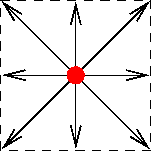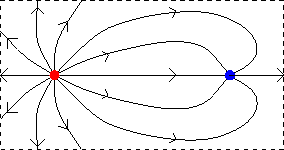Electric field lines

Electric field lines provide a means to visualize the electric field. Since the electric field is a vector, electric field lines have arrows showing the direction of the electric field. As two examples, we show the electric field lines of a single point charge, and of a positive and negative charge.The following rules apply to electric field lines:

1. Lines begin and end only at charges (beginning at + charges, ending at - charges) or at Infinity.

2. Lines are closer together where the field is stronger.

3. Larger charges have more field lines beginning or ending on them.

4. Electric Field lines never cross (since E must point in a definite direction unless it is zero).

5. At any location, the direction of the electric field is tangent to the electric field line that passes through that location.

For instance, the positive charge is stronger than the negative charge on the upper right diagram, since there are more lines originating from the positive charge and the lines from the negative charge are more strongly bent than the lines from the positive charge.